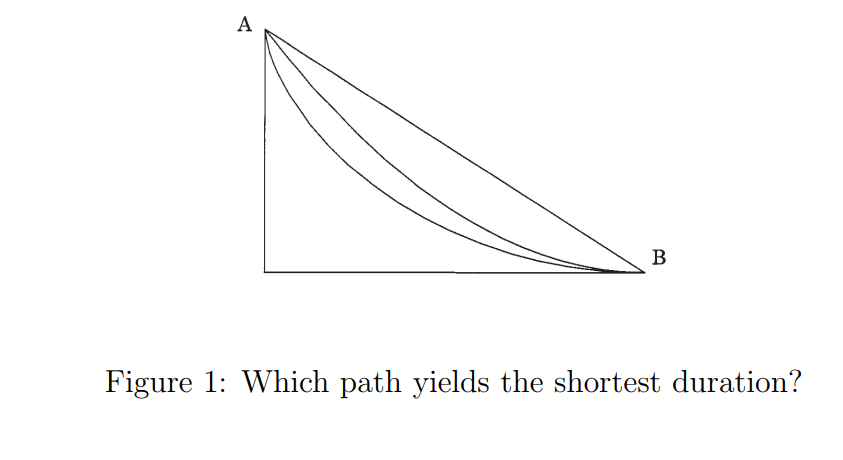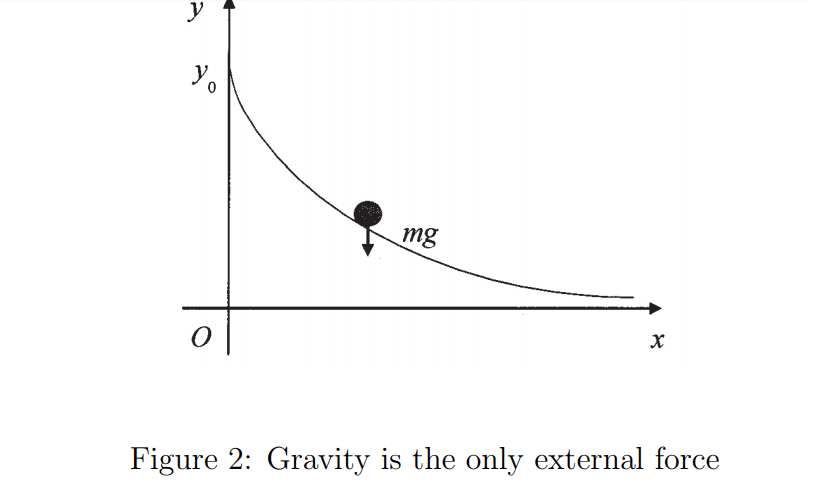# Brachistochrone Problem & cycloid. GIF: Source is Vsauce /…Brachistochrone Problem & cycloid.

GIF: Source is Vsauce / The Brachistochrone : https://www.youtube.com/watch?v=skvnj67YGmw.

Which is the quickest path? …. The cycloidis is the curve which yields the quickest descent.

Suppose there is an incline such as that shown in Figure 1. When a ball rolls from A to B, which curve yields the shortest duration? Let’s assume that we have three hypotheses: a straight line, a quadratic, and a cycloid. The shortest path from A to B is the straight line, so one might think that the straight path is the fastest, but in fact it is surprisingly slow. It’s better to select a path which has a downward drop in order to accelerate the ball in the first phase, so that it rolls quickly. The ball arrives earlier on the quadratic path than on the straight line path. However, increasing the degree of the function causes the ball to travel more slowly on the flat section.

It is said that Galileo (1564-1642) first presented this problem. It is also known that the cycloid is the curve which yields the quickest descent. This time I will discuss this problem, which may be handled under the field known as the calculus of variations, or variational calculus in physics, and introduce the charming nature of cycloid curves.